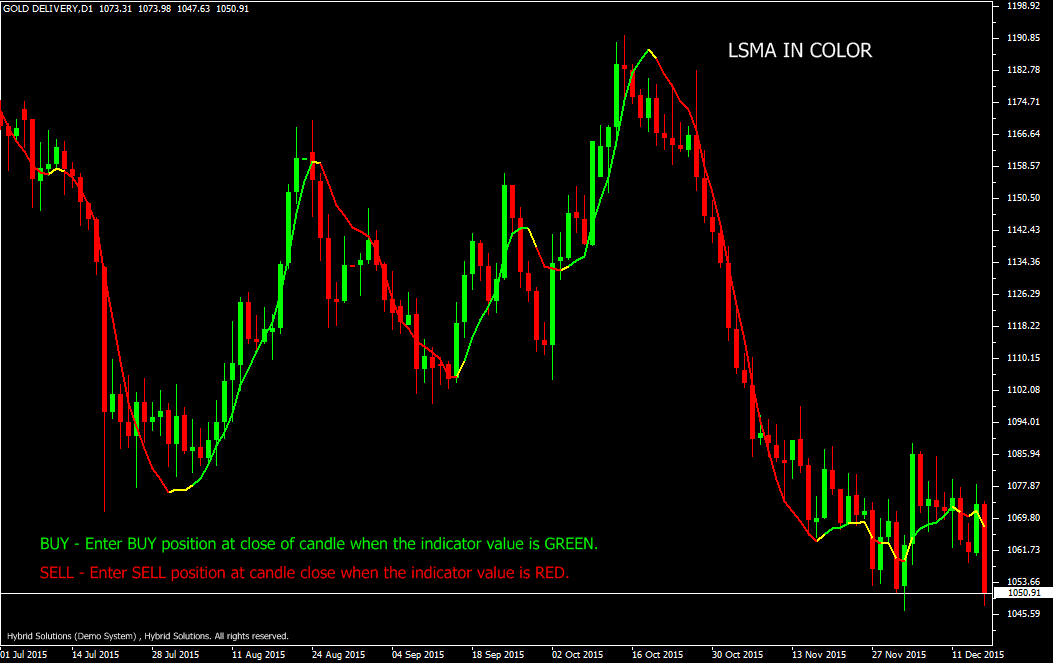# LSMA

Free

Developed By: NeeduVerdeSolution

In stock

LSMA in Color (Least Squares Moving Average) is a powerful VertexFX client side VTL indicator that provides adaptive Moving Average based on the statistical Least Squares fit algorithm. The LSMA in Color indicator requires at least 2 X R_PERIOD + 5 bars. For example, if R_PERIOD is 10, then the indicator requires 25 bars to construct the indicator.

SKU: 902 Categories: ,LSMA in Color (Least Squares Moving Average) is a powerful VertexFX client side VTL indicator that provides adaptive Moving Average based on the statistical Least Squares fit algorithm. The LSMA in Color indicator requires at least 2 X R_PERIOD + 5 bars. For example, if R_PERIOD is 10, then the indicator requires 25 bars to construct the indicator.

The concept behind the indicator is that price bars can be expressed as a linear or polynomial equation. The equation with the least error-fit (least squares regression fit) is the best representative of that indicator. It can be used to predict the next bar assuming that it is the best equation to represent the price bars over the recent R_PERIOD. At first step we calculate the regression error over the recent R_PERIOD bars. This value is then compared with the previous value. If it is lesser than the previous value, it implies that our equation for the line is better than the previous and this value is the new indicator value. When the price closes above the indicator value, the indicator color is set to Green and when the price closes below the indicator value, the indicator color is set to Red.

Buy: Place Buy position at close of candle when the indicator color is Green.
Do not open Buy position if candle has gap-up opening.
Stop Loss can be placed below the nearest swing low.

Sell: Place Sell position at candle close when the indicator value is Red.
Do not open Sell position if candle has gap-down opening.
Stop Loss can be placed above the nearest swing low.